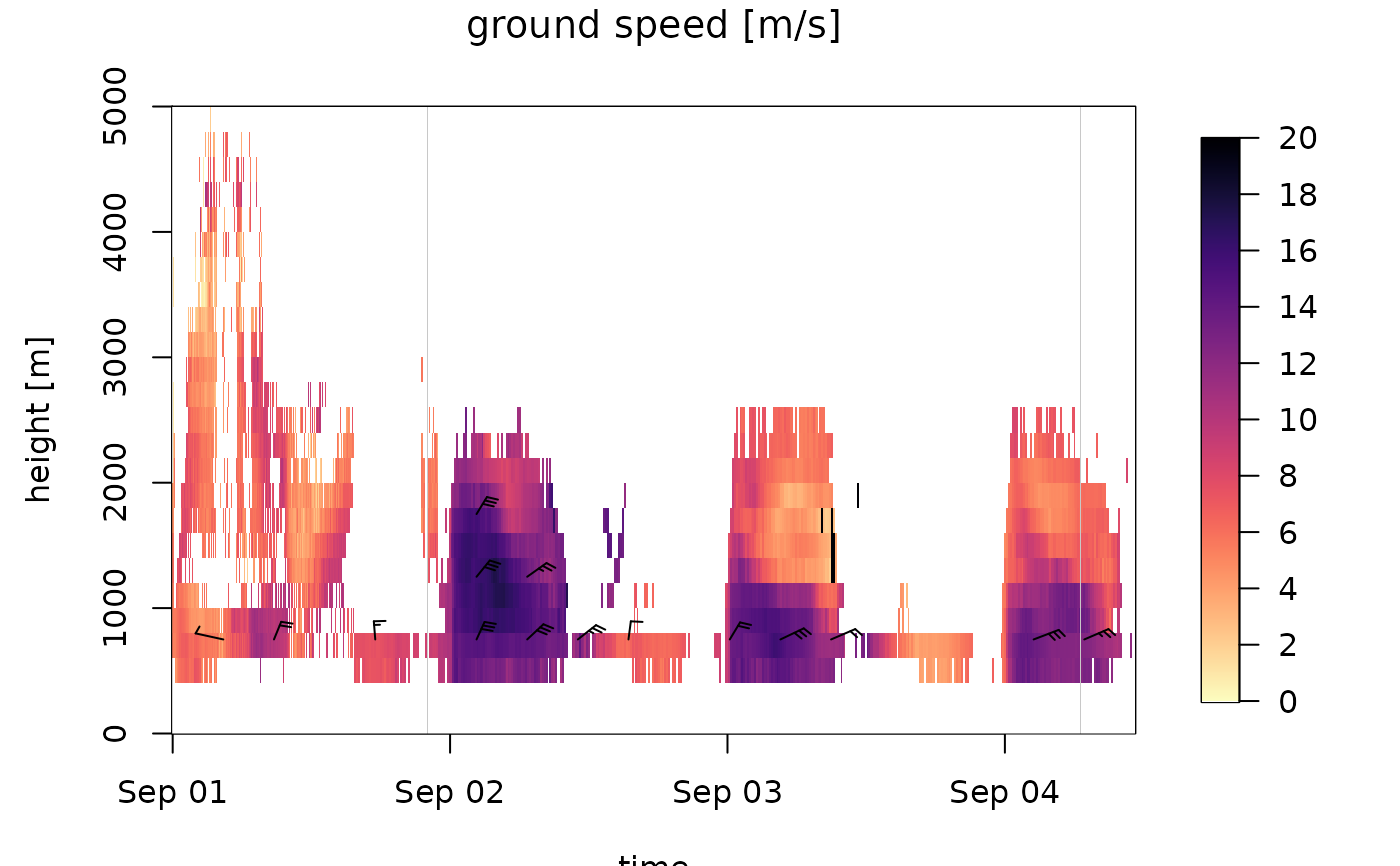Plot a time series of vertical profiles of class vpts.

# S3 method for vpts
plot(
x,
xlab = "time",
ylab = "height [m]",
quantity = "dens",
log = NA,
barbs = TRUE,
barbs_height = 10,
barbs_time = 20,
barbs_dens_min = 5,
zlim,
legend_ticks,
legend.ticks,
main,
barbs.h = 10,
barbs.t = 20,
barbs.dens = 5,
na_color = "#C8C8C8",
nan_color = "white",
n_color = 1000,
palette = NA,
...
)

## Arguments

x

A vp class object inheriting from class vpts.

xlab

A title for the x-axis.

ylab

A title for the y-axis.

quantity

Character string with the quantity to plot, one of 'dens','eta','dbz','DBZH' for density, reflectivity, reflectivity factor and total reflectivity factor, respectively.

log

Logical, whether to display quantity data on a logarithmic scale.

barbs

Logical, whether to overlay speed barbs.

barbs_height

Integer, number of barbs to plot in altitudinal dimension.

barbs_time

Integer, number of barbs to plot in temporal dimension.

barbs_dens_min

Numeric, lower threshold in aerial density of individuals for plotting speed barbs in individuals/km^3.

zlim

Optional numerical atomic vector of length 2, specifying the range of quantity values to plot.

legend_ticks

Numeric atomic vector specifying the ticks on the color bar.

legend.ticks

main

A title for the plot.

barbs.h

barbs.t

barbs.dens

na_color

Color to use for NA values, see class vpts conventions.

nan_color

Color to use for NaN values, see class vpts conventions.

n_color

The number of colors (>=1) to be in the palette.

palette

(Optional) character vector of hexadecimal color values defining the plot color scale, e.g. output from viridis

...

Additional arguments to be passed to the low level image plotting function.

## Details

Aerial abundances can be visualized in four related quantities, as specified by argument quantity:

"dens"

the aerial density of individuals. This quantity is dependent on the assumed radar cross section (RCS) in the x$attributes$how\$rcs_bird attribute

"eta"

reflectivity. This quantity is independent of the value of the rcs_bird attribute

"dbz"

reflectivity factor. This quantity is independent of the value of the rcs_bird attribute, and corresponds to the dBZ scale commonly used in weather radar meteorology. Bioscatter by birds tends to occur at much higher reflectivity factors at S-band than at C-band

"DBZH"

total reflectivity factor. This quantity equals the reflectivity factor of all scatterers (biological and meteorological scattering combined)

Aerial velocities can be visualized in three related quantities, as specified by argument quantity:

"ff"

ground speed. The aerial velocity relative to the ground surface in m/s.

"u"

eastward ground speed component in m/s.

"v"

northward ground speed component in m/s.

### barbs

In the speed barbs, each half flag represents 2.5 m/s, each full flag 5 m/s, each pennant (triangle) 25 m/s

### legend_ticks / zlim

Default legend ticks and plotting range are specified based on quantity, radar wavelength (S- vs C-band), and value of log

### log

Quantities u and v cannot be plotted on a logarithmic scale, because these quantities assume negative values. For quantities DBZH and dbz log=TRUE is ignored, because these quantities are already logarithmic.

## Examples

# locate example file:
ts <- example_vpts
# plot density of individuals for the first 500 time steps, in the altitude
# layer 0-3000 m.
plot(ts[1:500], ylim = c(0, 3000))
#> Warning: Irregular time-series: missing profiles will not be visible. Use 'regularize_vpts' to make time series regular.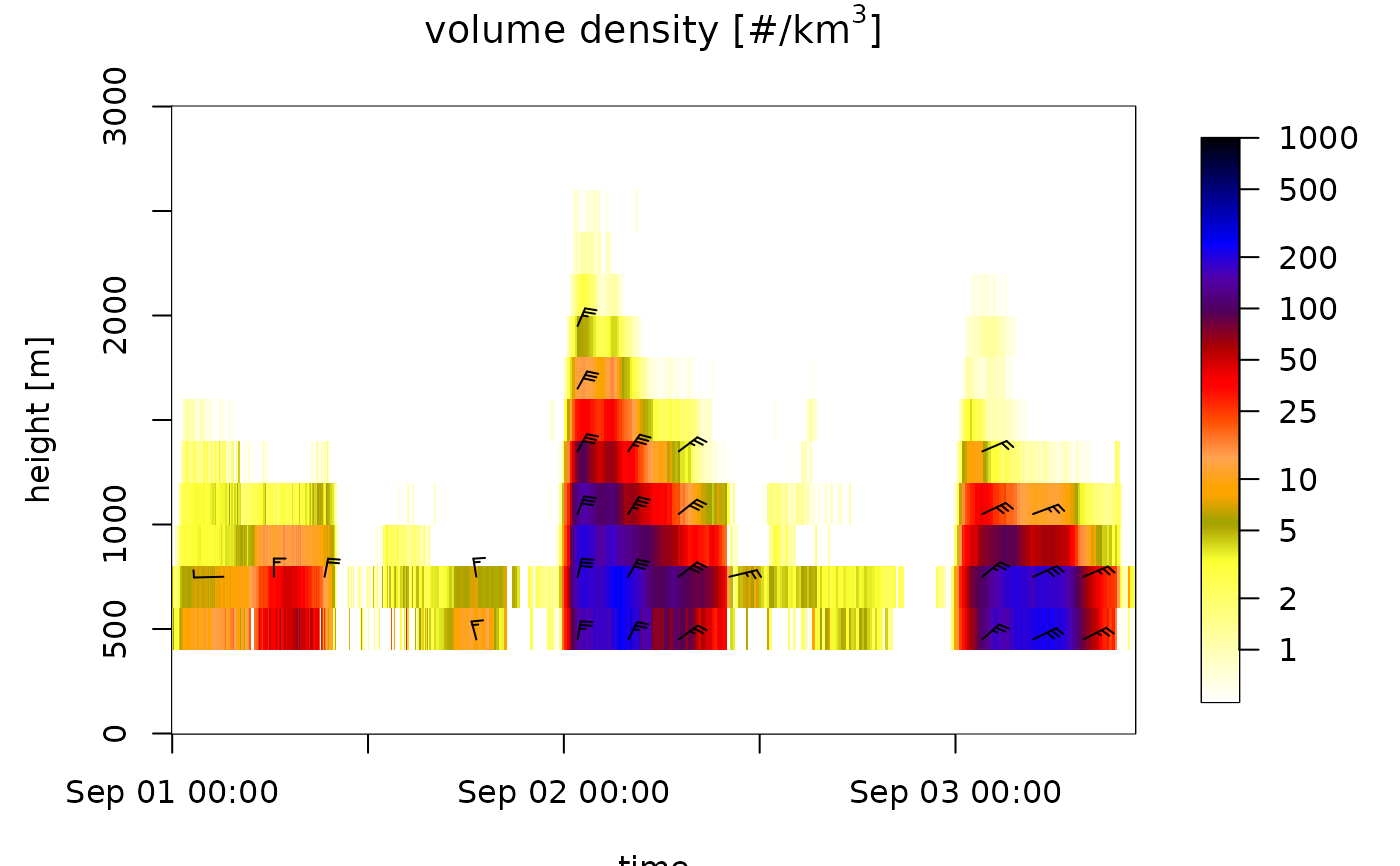# plot total reflectivity factor (rain, birds, insects together):
plot(ts[1:500], ylim = c(0, 3000), quantity = "DBZH")
#> Warning: Irregular time-series: missing profiles will not be visible. Use 'regularize_vpts' to make time series regular.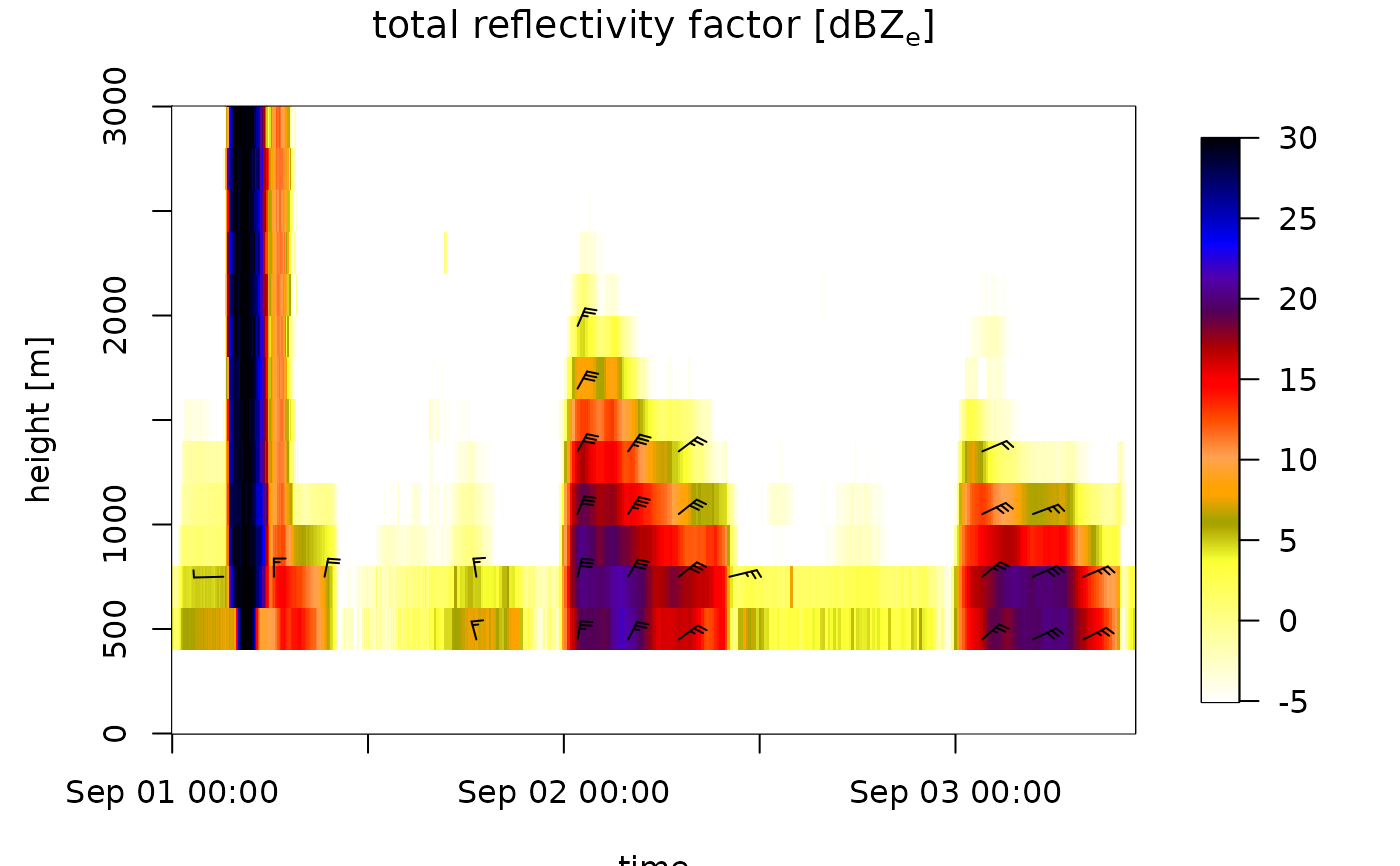# regularize the time grid, which includes empty (NA) profiles at
# time steps without data:
ts_regular <- regularize_vpts(ts)
#> projecting on 300 seconds interval grid...
plot(ts_regular)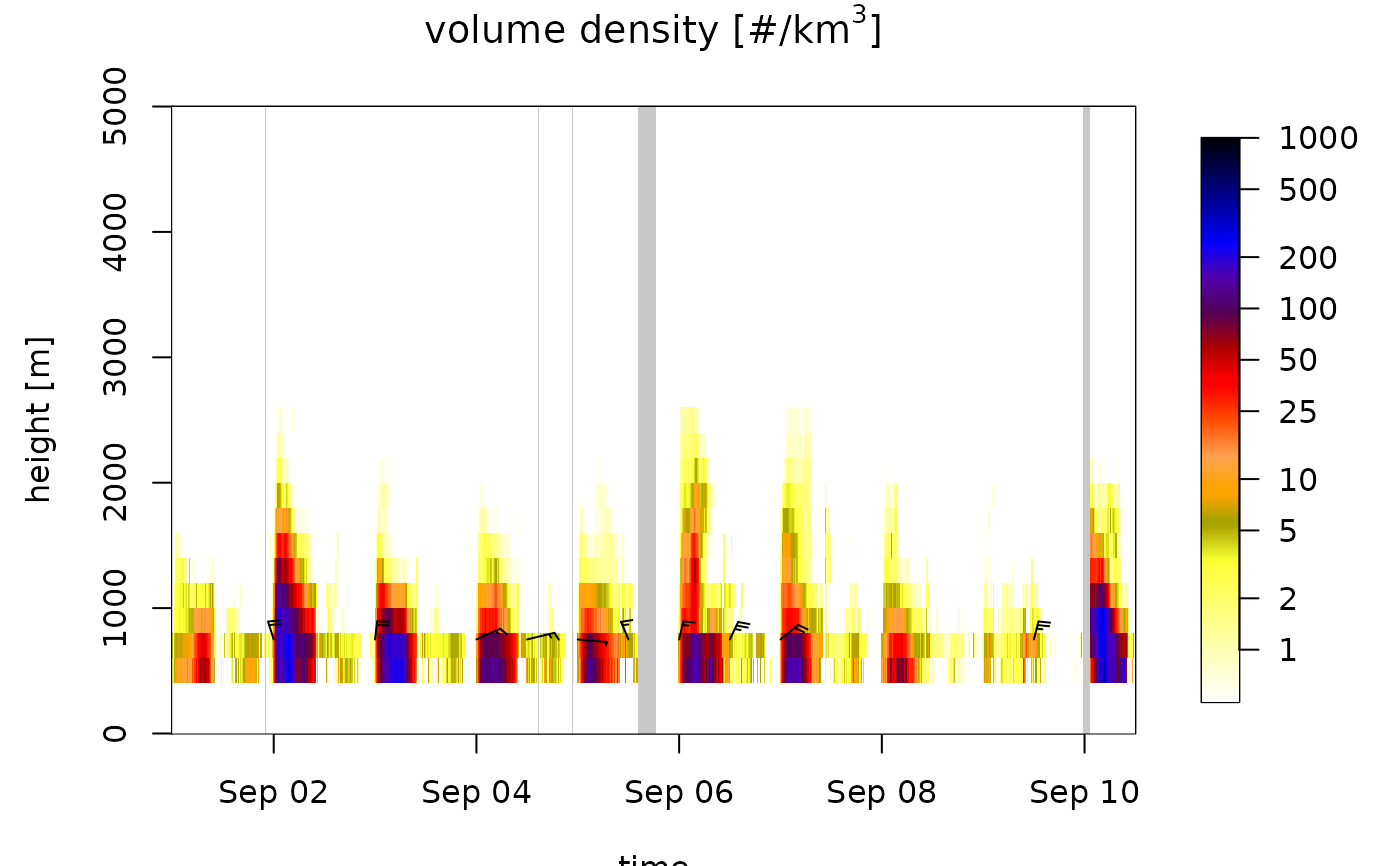# change the color of missing NA data to red
plot(ts_regular, na_color="red")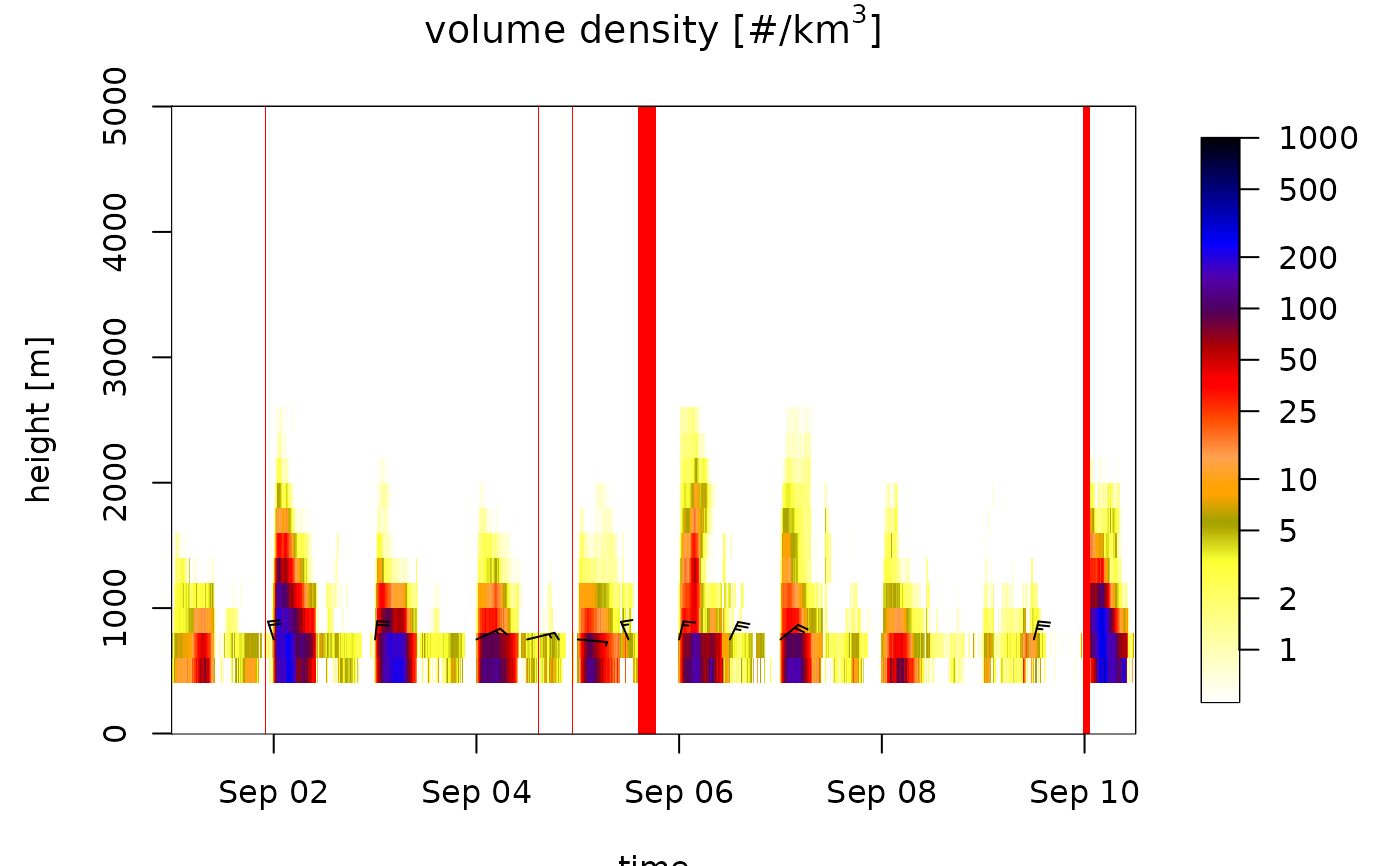# change the color palette:
plot(ts_regular[1:1000], ylim = c(0, 3000), palette=viridis::viridis(1000))# change and inverse the color palette:
plot(ts_regular[1:1000], ylim = c(0, 3000), palette=rev(viridis::viridis(1000, option="A")))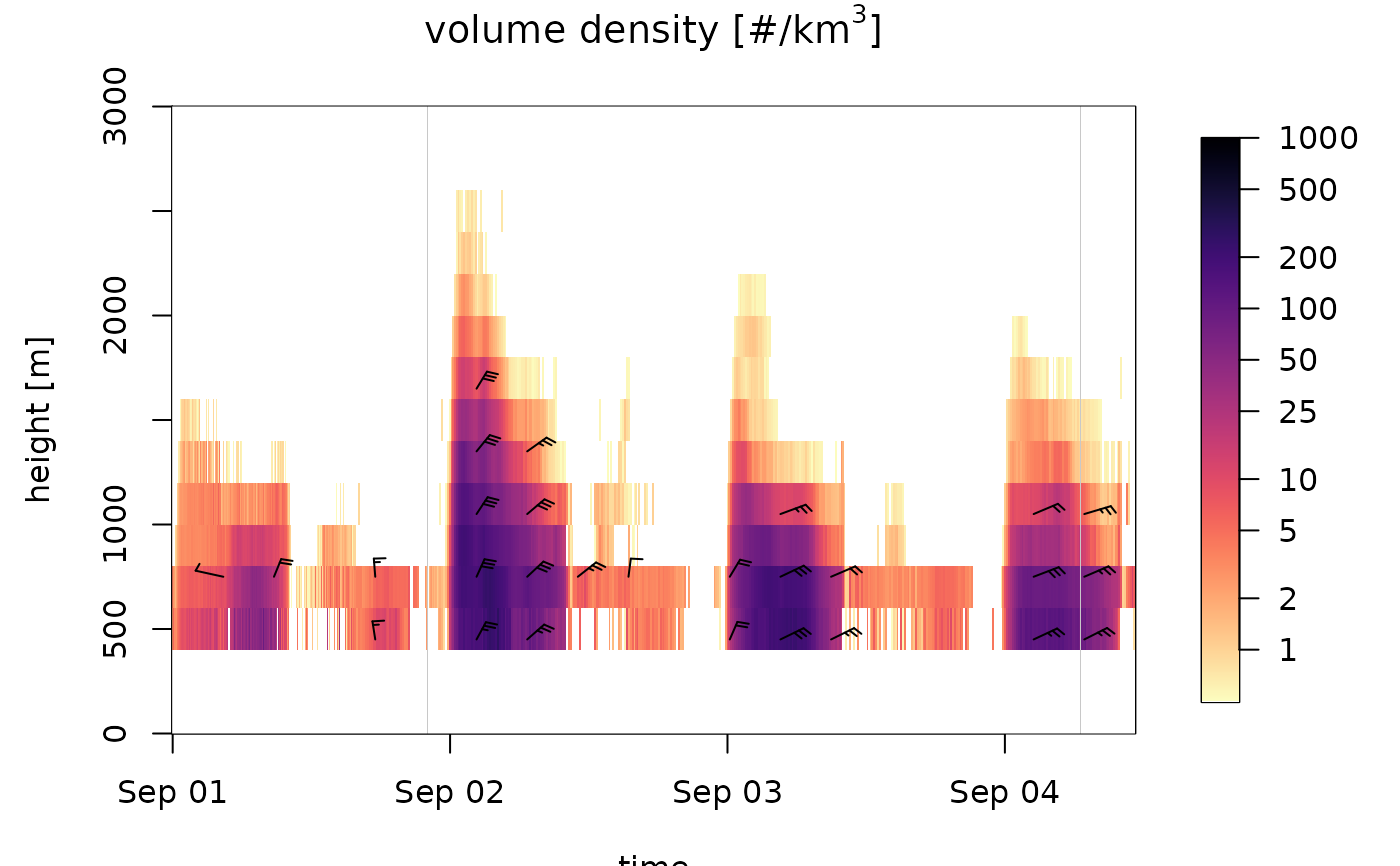# plot the speed profile:
plot(ts_regular[1:1000], quantity="ff")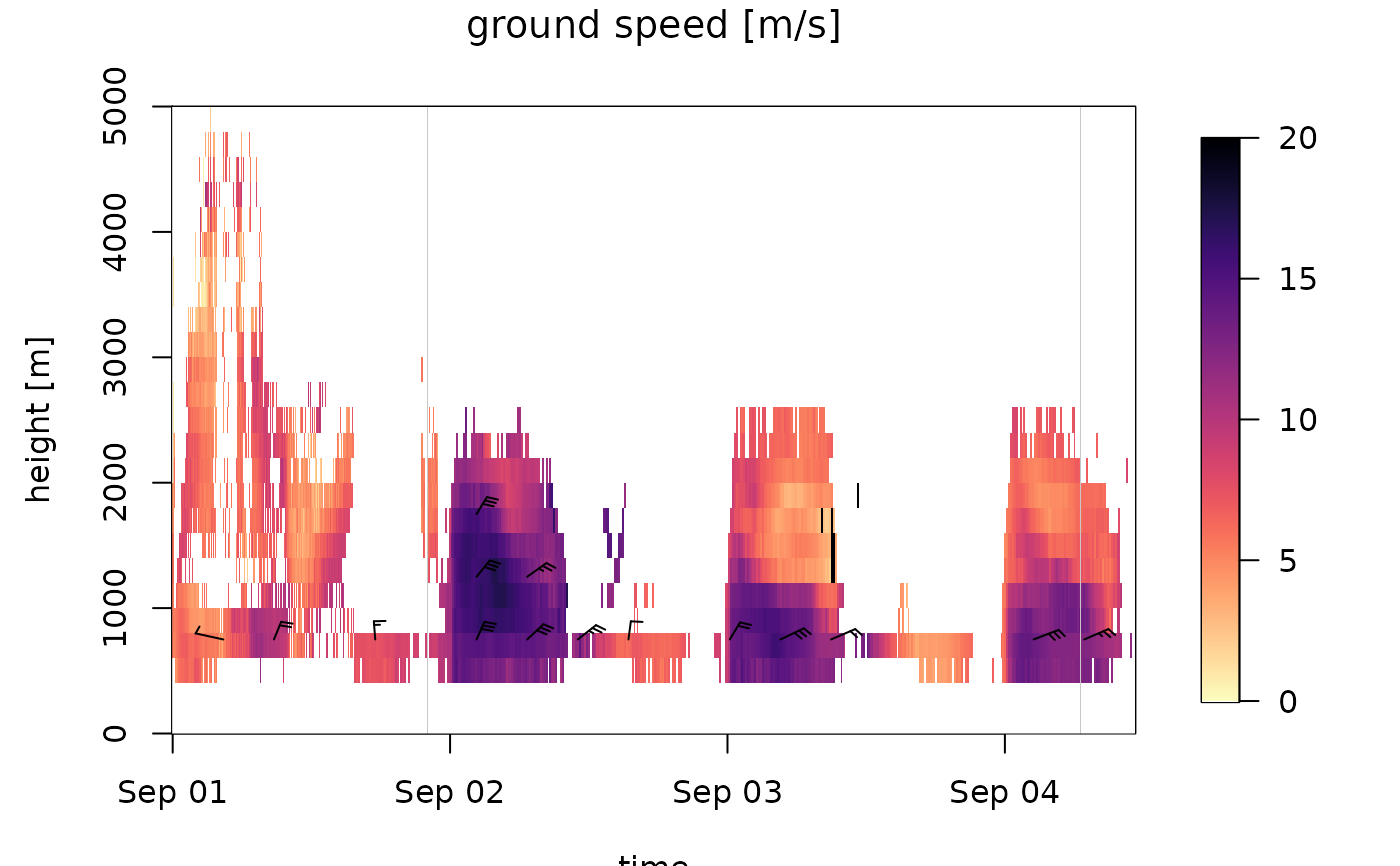# plot the northward speed component:
plot(ts_regular[1:1000], quantity="v")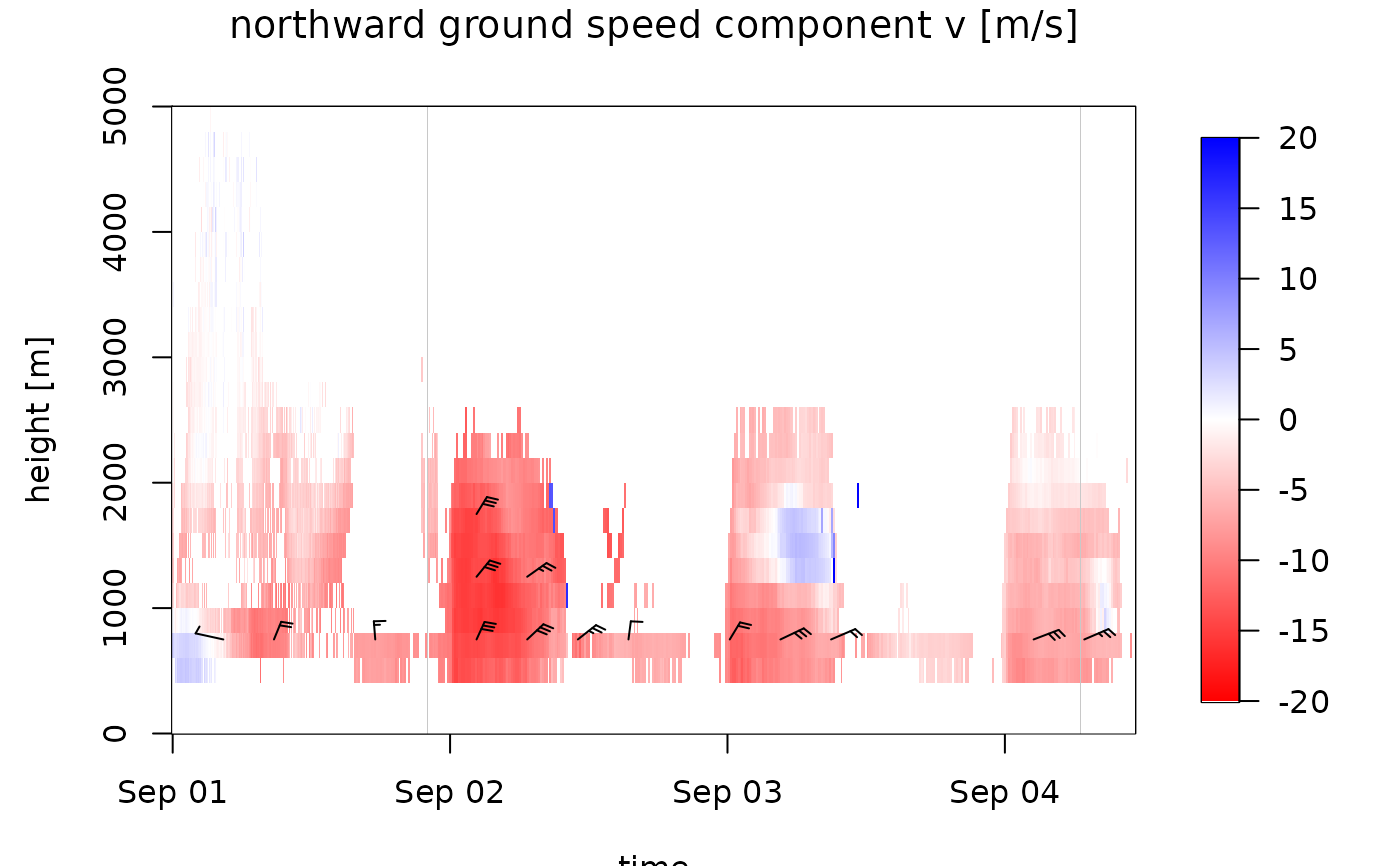# plot speed profile with more legend ticks,
plot(ts_regular[1:1000], quantity="ff", legend_ticks=seq(0,20,2), zlim=c(0,20))